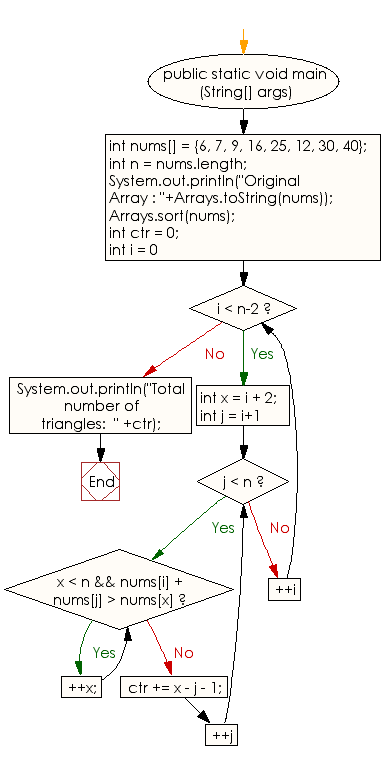﻿ Java: Find the number of triangles in an unsorted array# Java Array Exercises: Count the number of possible triangles from a given unsorted array of positive integers

## Java Array: Exercise-44 with Solution

Write a Java program to count the number of possible triangles from a given unsorted array of positive integers.

Note: The triangle inequality states that the sum of the lengths of any two sides of a triangle must be greater than or equal to the length of the third side.

Sample Solution:

Java Code:

``````import java.util.*;
import java.lang.*;
public class Main
{
public static void main (String[] args)
{
int nums[] = {6, 7, 9, 16, 25, 12, 30, 40};
int n = nums.length;
System.out.println("Original Array : "+Arrays.toString(nums));

// Sort the array elements in non-decreasing order
Arrays.sort(nums);

// Initialize count of triangles
int ctr = 0;

for (int i = 0; i < n-2; ++i)
{
int x = i + 2;

for (int j = i+1; j < n; ++j)
{
while (x < n && nums[i] + nums[j] > nums[x])
++x;
ctr += x - j - 1;
}
}
System.out.println("Total number of triangles:  " +ctr);
}
}
```
```

Sample Output:

```
Original Array : [6, 7, 9, 16, 25, 12, 30, 40]
Total number of triangles:  17
```

Flowchart:Visualize Java code execution (Python Tutor):

Java Code Editor:

Improve this sample solution and post your code through Disqus

What is the difficulty level of this exercise?

Test your Programming skills with w3resource's quiz.

﻿

## Java: Tips of the Day

countOccurrences

Counts the occurrences of a value in an array.

Use Arrays.stream().filter().count() to count total number of values that equals the specified value.

```public static long countOccurrences(int[] numbers, int value) {
return Arrays.stream(numbers)
.filter(number -> number == value)
.count();
}
```

Ref: https://bit.ly/3kCAgLb